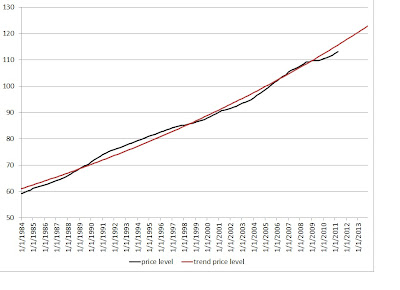## Sunday, September 11, 2011

### Inflating Away Debts

On Coordination Problem, Peter Boettke is skeptical about Rogoff's suggestion that 4% to 5% inflation for a decade would be desirable because it would reduce the real value of debt. Boettke suggests that Rajan's criticisms of Rogoff are more appropriate.

While I agree that shifting to a new inflation target for a decade would be undesirable, I think returning the price level to its growth path of the Great Moderation would be justified. To return to that growth path over the next year would require an inflation rate of 4.7%. Of course, to remain on that growth path, the inflation rate would then return to 2.4%. That is hardly 4% to 5% inflation for a decade.

The diagram below shows the price level measured using the GDP deflator and its trend from the Great Moderation.I favor nominal GDP targeting. When there are adverse productivity shocks, the price level moves to a higher growth path, which results in higher inflation. According to the Congressional Budget Office, the U.S. economy has suffered a productivity slowdown since 2000.

In the diagram below, the blue line shows the growth rate of potential output estimated by the CBO. The red line shows the 3% trend growth rate of real GDP (and real potential GDP) from the Great Moderation.With nominal GDP targeting, the productivity slowdown will result in higher inflation. Using the trend growth path of nominal GDP from the Great Moderation, and dividing by the CBO estimate of GDP, the resulting GDP deflator would imply substantially higher inflation since 2001.

In the diagram below, inflation rises above the 2.4% trend in 2000, rises to 3% in 2005 and peaks at nearly 4% in 2009. Even in 2011, the inflation rate consistent with nominal GDP remaining on its growth path of the Great Moderation would be 3%.Of course, in reality, the Fed allowed nominal GDP to fall far below that growth path. If nominal GDP was returned to its growth path of the Great Moderation, and real GDP returned to the CBO estimate of potential output, then the price level, shown as P** in the diagram below, would be 6% higher than the trend of the Great Moderation. All of those quarters with inflation greater than 2.4% add up.The current price level is 8% below the level consistent with nominal GDP targeting--assuming the CBO estimate of the productivity slowdown is correct. For the price level to return to that level over the next year, the inflation rate would need to be 12.6%.

It would be possible to adjust to that growth path over a longer time horizon. The CBO provides estimates of potential output until 2020, and 5% inflation for 5 years would result in a GDP deflator of 144.3, very close to the GDP trend value from the Great Moderation of \$22.9 trillion for the second quarter of 2016 divided by the CBO estimate of potential output of \$15.9 trillion.

With nominal GDP targeting, after this gradual catch up, the inflation rate would need to slow. However, the CBO expects the potential output slowdown to continue through 2016, so that inflation would remain 3%.

1.2.